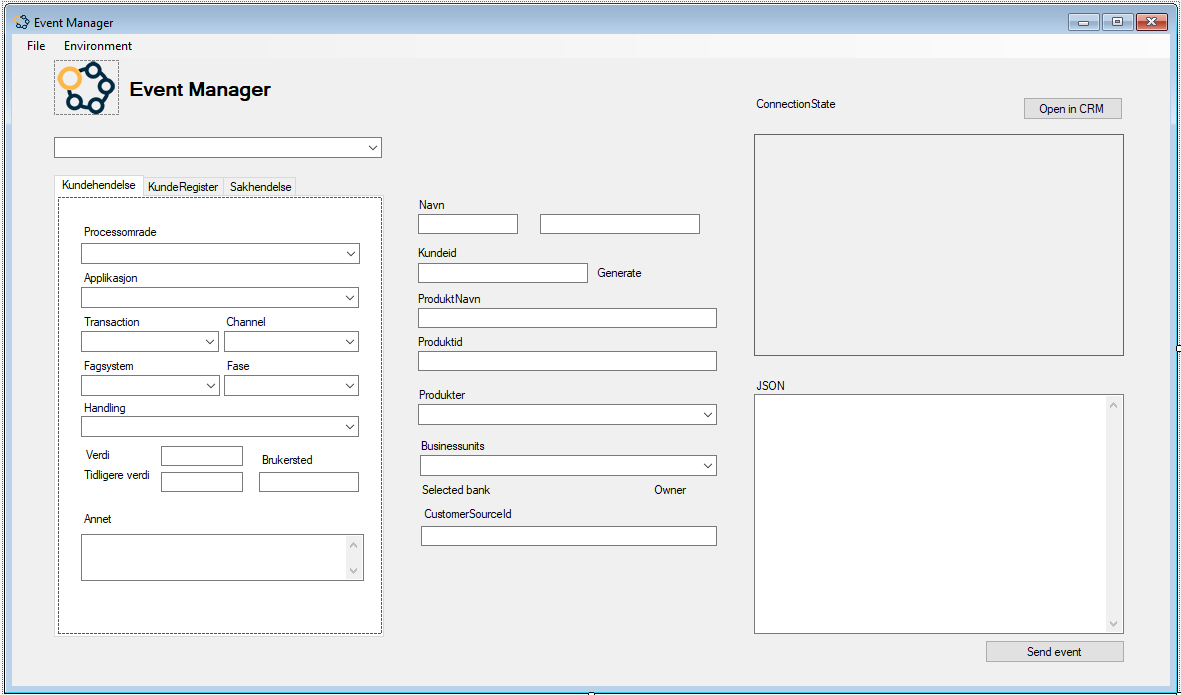# Generate a valid Norwegian SSN

I was facing a test project where I needed to generate test customers for the client in C#. Since I'm lazy and like to create dynamic functions, I needed to create a function that could return a customer with a valid, but though fake SSN(Social security number), the gender, a fore and lastname. I started to search Google, there must be someone who actually already accomplished this function, - Ohh, none had acheived this yet. I needed to create this function on my own, because I really wanted to make the testing for my customer as easy as possible.

## Event Manager

Below is a screenshot of the event manager that creates the event and send the event as a JSON to create a new record in Dynamics CRM with the business rules applied.## Generate SSN

See the code below. Full code is located at my space on Bitbucket.

I dicovered that there was a function in a Wiki, that explains how to calculate if its a valid SSN. Hum, I needed to reverse the function, and this is the code that I came up with.

``````public static partial class Extensions
{
/// <summary>
/// Function to create a fake but valid Norwegian SSN
/// </summary>
/// <returns>A valid SSN object with SSN number, name, lastname and gender</returns>
public static SSN GenSSN()
{
//Random date
Func<DateTime> RandomDateFunc()
{
var start = new DateTime(1900, 1, 1); // Little bit optmistic by year, anyhow... = 119 years old!!
var gen = new Random();
int range = ((TimeSpan)(DateTime.Today - start)).Days;
}

var rex = new Regex(@"^(?<birthdate>(?<day>dd)(?<month>dd)(?<year>dd))(?<individual>dd(?<gender>d))(?<checksum>dd)\$");
var yyyy = RandomDateFunc().Invoke();
string formatOut = "ddMMyy";
var date = yyyy.ToString(formatOut);

int d1 = Convert.ToInt32(date.Substring(0, 1));
int d2 = Convert.ToInt32(date.Substring(1, 1));
int m1 = Convert.ToInt32(date.Substring(2, 1));
int m2 = Convert.ToInt32(date.Substring(3, 1));
int y1 = Convert.ToInt32(date.Substring(4, 1));
int y2 = Convert.ToInt32(date.Substring(5, 1));
int c1 = 0, c2 = 0, i1 = 0, i2 = 0, i3 = 0;

do{ //Find mathing pairs of c1 and c2
//c2 need to be equal to last digit.
var r = new Random();
var num = r.Next(100, 499); //Wrong but here we dont need perfect numbers since all are fake.
int[] n = num.ToString().ToCharArray().Select(c => Convert.ToInt32(c.ToString())).ToArray();
i1 = n; i2 = n; i3 = n;

//First num (See wiki)
var v1 = (3 * d1) + (7 * d2) + (6 * m1) + m2 + (8 * y1) + (9 * y2) + (4 * i1) + (5 * i2) + (2 * i3);
c1 = (v1 % 11) == 0 ? 0 : 11 - (v1 % 11);

//Second/last num (See wiki)
int v2 = (5 * d1) + (4 * d2) + (3 * m1) + (2 * m2) + (7 * y1) + (6 * y2) + (5 * i1) + (4 * i2) + (3 * i3) + (2 * c1);
c2 = (v2 % 11) == 0 ? 0 : 11 - (v2 % 11);

//Do until c1 and c2 is lower than 10 and both are true.
if ((c1 < 10) && (c2 < 10))
break;

} while (true);

var ssn = \$"{d1}{d2}{m1}{m2}{y1}{y2}{i1}{i2}{i3}{c1}{c2}";
var match = rex.Match(ssn);
var gender = int.Parse(match.Groups["gender"].Value);
var sex = gender % 2 == 0 ? "Male" : "Female"; // If wanted
//Console.WriteLine(sex);
var s = new SSN(sex){ Number = ssn, Gender = sex };

return s;
}

}

/// <summary>
/// Holder of a Person data
/// </summary>
public class SSN
{
public string Number { get; set; }
public string Gender { get; set; }

public string FirstName { get; private set; }

public string LastName { get; private set; }

//<----- Thousends of combinations of names.. See code on my space on bitbucket

public string FirstNameMale = @"Jan,Per,Bjørn,Ole,Lars,Kjell,Knut";
public string FirstNameFemale = @"Anne,Inger,Kari,Marit,Ingrid,Liv,Eva";
public string LastNames = @"Hansen,Johansen,Olsen,Larsen";

/// <summary>
/// Creates a new instance of SSN
/// </summary>
/// <param name="gender">The gender to generate.</param>
public SSN(string gender)
{
var rand = new Random();

if (gender == "Male")
FirstName = FirstNameMale.Split(',')[rand.Next(0,49)];
if (gender == "Female")
FirstName = FirstNameFemale.Split(',')[rand.Next(0, 49)];

LastName = LastNames.Split(',')[rand.Next(0, 3438)];
}
}``````

Created by Bootproject editor on 2019-10-18

•